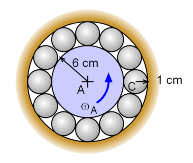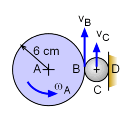Ch 5. Rigid Body General Motion Multimedia Engineering Dynamics Fixed Axis Rotation Plane Motion Velocities Zero Velocity Point Plane Motion Accelerations Multiple Gears Rot. Coord. Velocities Rot. Coord. Acceleration
 Chapter - Particle - 1. General Motion 2. Force & Accel. 3. Energy 4. Momentum - Rigid Body - 5. General Motion 6. Force & Accel. 7. Energy 8. Momentum 9. 3-D Motion 10. Vibrations Appendix Basic Math Units Basic Equations Sections Search eBooks Dynamics Fluids Math Mechanics Statics Thermodynamics Author(s): Kurt Gramoll ©Kurt GramollDYNAMICS - EXAMPLERotating Shaft with Ball Bearings Example A cylindrical shaft is supported by spherical ball bearings that freely rotate. There is no slipping between the center cylinder and the bearings, or between the bearings and the outside surface. If the cylinder rotates at 4 rad/s, what is the velocity magnitude of a ball bearing center (point C)? SolutionShaft and Bearing Orientation At any given instant, both points A and D are not moving. Thus, use those two points as pinned or fixed points (of course, an instant later, point D will be at a different location). Since there is no slipping, the velocity of point B will be the same on shaft or ball, thus,      vB = ωA rAB = ωC rBD      4(6) = ωC (2)      ωC (2) = 12 rad/s Now point C can be found by using the velocity of point D and B.      vC = vD + vC/D = 0 + ωC × rDC      vC = ωC rDC          = 12 (1) = 12 cm/s vC = 12 cm/s

Practice Homework and Test problems now available in the 'Eng Dynamics' mobile app
Includes over 400 problems with complete detailed solutions.
Available now at the Google Play Store and Apple App Store.